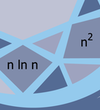# Computational Complexity of Polynomial Time Problems#### This series of talks was part of the Fine-Grained Complexity and Algorithm Design Boot Camp. Videos for each talk area available through the links above.

A central goal of theoretical computer science is the classification of computational problems according to their complexity. The theory of NP-hardness has been successful in identifying problems that are unlikely to be solvable in time that is polynomial in the size of the input. Conversely, for many problems of practical interest, the field has developed intriguingly fast polynomial time solutions. However, improving the running times of many of these algorithms has been a longstanding open problem, with little to no progress. It is thus completely plausible that these algorithms are optimal. Showing that this is indeed the case would be a great accomplishment of the theory of algorithms.

Unfortunately, unconditional lower bounds seem hard to obtain. Instead, an alternative approach for proving hardness of problems in P is to identify plausible complexity-theoretic conjectures and show that the currently best algorithms for the problems of interest are optimal if one assumes these conjectures. Such popular conjectures include the (Strong) Exponential Time Hypothesis (that concerns the complexity of CNF-SAT), the conjecture that all-pairs shortest paths (APSP) requires essentially cubic time, or that 3SUM requires essentially quadratic time.

The goal of the minicourse is to expose the audience to the recent growing body of conditional lower bounds for a variety of problems in diverse areas, such as graph optimization, string matching, geometry, and dynamic data structures, and to the attempts at unifying and/or refuting the popular conjectures.